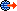# Tutorial 3: Using Matrices

The Matrices tutorial project introduces the concept of matrices and shows how to use them. Matrices are used to transform the coordinates of vertices and to set up cameras and viewports.

## Path

Source location: (SDK root)\Samples\Managed\Direct3D\Tutorials\Tutorial3

## Procedure

Note: For information about initializing Microsoft Direct3D, handling Microsoft Windows messages, rendering, or shutting down, see Tutorial 1: Creating a Device.

Tutorial 2: Rendering Vertices rendered 2-D vertices to draw a triangle. This tutorial adds to the code of Tutorial 2 to rotate the triangle using 3-D vertex transformations.

Because this project will apply transformations to the triangle object, instead of using already-transformed 2-D window coordinates as in Tutorial 2, the vertex buffer is initialized with the CustomVertex.CustomVertex.PositionColored structure, as in the following code fragment.

``````          [C#]

Device dev = (Device)sender;
// Now create the vertex buffer.
vertexBuffer = new VertexBuffer(typeof(CustomVertex.PositionColored),
3,
dev,
0,
CustomVertex.PositionColored.Format,
Pool.Default);
``````

In addition, within the private Render method, the device vertex format is initialized to be the CustomVertex.PositionColored format, as shown in the following code. Before geometry is rendered, the application-defined SetupMatrices method, which creates and sets the 3-D matrix transformations of the triangle object, is called from Render.

``````          [C#]

private void Render()
{
.
.
.
// Set up the world, view, and projection matrices.
SetupMatrices();

device.SetStreamSource(0, vertexBuffer, 0);
device.VertexFormat = CustomVertex.PositionColored.Format;
device.DrawPrimitives(PrimitiveType.TriangleList, 0, 1);

// End the scene.
device.EndScene();
device.Present();
}
``````

Typically, three types of transformation are set for a 3-D scene. The transformations are all defined as properties of the Transforms object, accessed from the Device.Transform property. All use a left-handed coordinate system typical of Direct3D; see 3-D Coordinate Systems.

1. World Transformation Matrix: In this case, the triangle is rotated around the y-axis by calling the Matrix.RotateY method, as shown in the following code sample. Note that Matrix is part of the general-purpose Microsoft.DirectX namespace. This call uses the system Environment.TickCountmethod, divided by a scaling value, to provide the RotateY argument in radians. This procedure yields a smoothly-varying rotation about the y-axis.
2. View Transformation Matrix: The view transformation matrix yields the camera view of the scene, in this sample code by calling the Matrix.RotateY method. Three Vector3 vectors form the arguments for the LookAtLH method, which builds a left-handed (LH) look-at matrix. The three vectors represent respectively the eye location, the camera look-at target (in this case the origin), and the current world's up-direction.
3. Projection Transformation Matrix: The projection transformation matrix defines how geometry is transformed from 3-D view space to 2-D viewport space. In this sample code it is formed from the matrix returned by the left-handed PerspectiveFovLH method. Arguments to the method are the field of view in radians, the aspect ratio (view space height divided by width), the near clipping plane distance, and the far clipping plane distance.

The order in which these transformation matrices are created does not affect the layout of the objects in a scene. However, Direct3D applies the matrices to the scene in the above order.

``````          [C#]

private void SetupMatrices()
{
// For our world matrix, we will just rotate the object about the y-axis.

// Set up the rotation matrix to generate 1 full rotation (2*PI radians)
// every 1000 ms. To avoid the loss of precision inherent in very high
// floating point numbers, the system time is modulated by the rotation
// period before conversion to a radian angle.
int  iTime  = Environment.TickCount % 1000;
float fAngle = iTime * (2.0f * (float)Math.PI) / 1000.0f;
device.Transform.World = Matrix.RotationY( fAngle );

// Set up our view matrix. A view matrix can be defined given an eye
// point, a point to lookat, and a direction for which way is up. Here,
// we set the eye five units back along the z-axis and up three units,
// look at the origin, and define "up" to be in the y-direction.

device.Transform.View = Matrix.LookAtLH(
new Vector3( 0.0f, 3.0f,-5.0f ),
new Vector3( 0.0f, 0.0f, 0.0f ),
new Vector3( 0.0f, 1.0f, 0.0f ) );

// For the projection matrix, we set up a perspective transform (which
// transforms geometry from 3D view space to 2D viewport space, with
// a perspective divide making objects smaller in the distance). To build
// a perpsective transform, we need the field of view (1/4 pi is common),
// the aspect ratio, and the near and far clipping planes (which define
// at what distances geometry should be no longer be rendered).
device.Transform.Projection = Matrix.PerspectiveFovLH(
(float)Math.PI / 4,
1.0f,
1.0f,
100.0f );
}
``````

The character of rendering is controlled by setting properties of the RenderStateManager class. This is done in the OnResetDevice application-defined method, as shown in the following code fragment.

``````          [C#]

public void OnResetDevice(object sender, EventArgs e)
{
Device dev = (Device)sender;
// Turn off culling, so the user sees the front and back of the triangle
dev.RenderState.CullMode = Cull.None;
// Turn off Direct3D lighting, since object provides its own vertex colors
dev.RenderState.Lighting = false;
}
``````

In this case, culling beyond a backplane and Direct3D lighting are both turned off. These settings allow the full depth of the 3-D object to be viewed and the object to provide its own colors.